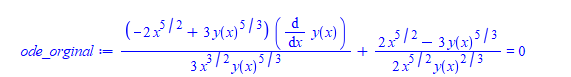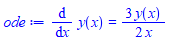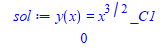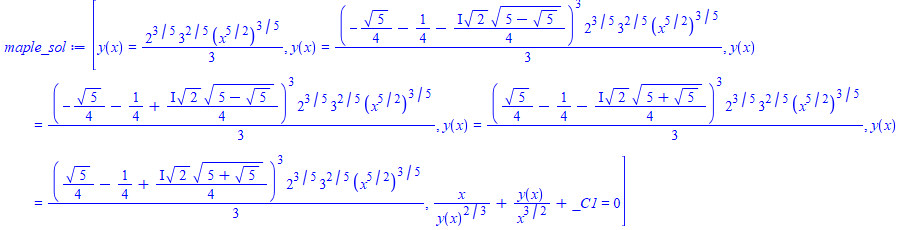# Question:why dsolve do not show this solution?

## Question:why dsolve do not show this solution?

Maple 2021

I was wondering why dsolve do not show this one solution I obtained different way, for this ode.

Maple gives 6 solutions to the ODE. But 5 of them are signular (have no constant of integration in them). I am looking at the last solution it gives, the one with constant of integration.

But when I solve this ODE, after simplifying it, I get different general solution than the last one Maple shows. I thought they might be equivalent, but I do not see how they could be.

So my question is why Maple did not show the simpler solution also?  Here is the code

`ode_orginal:=1/3*(-2*x^(5/2)+3*y(x)^(5/3))/x^(3/2)*diff(y(x),x)/y(x)^(5/3)+1/2*(2*x^(5/2)-3*y(x)^(5/3))/x^(5/2)/y(x)^(2/3) = 0;`This looks complicated and non-linear, but when I solve for y'(x) it gives much simpler and linear ODE (Have to convert the ODE to D before solving for diff(y(x),x), since Maple can complain otherwise).

```ode:=convert(ode_orginal,D);
ode:=diff(y(x),x)=solve(ode,D(y)(x),allsolutions=true);
```And solving the above gives the much simpler solution

```sol:=dsolve(ode,y(x));
odetest(sol,ode);```Now when using dsolve on the original complicated lookin ODE it gives

```maple_sol:=[dsolve(ode_orginal)];
```if there is any hope that one of the above 6 solutions will be the same as the simpler solution, it has to be the last one, with the _C1 in it, since all the others do not have _C1 (singular solutions).

But solving for y(x) from the last one does not give the simpler solution.

So it is a new general solution for the original ode and it is correct, since odetest gives zero.

I think in the process of solving for y'(x), some solutions got lost. Even though I asked for allsolutions there?.

But my main question is: Should dsolve have also have given the simpler solution? Since that one also satisfies the original nonlinear complicated ode

```odetest(sol,ode_orginal);
```

gives 0.

Maple 2021.1﻿